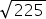Mathematics
Easy

Question

# Rewriteinto simplest form.

## 13141525Hint:

## The correct answer is: 15

### Here, we have write the simplest form of √225 .Now, √225 = √ ( 3 × 3 × 5 × 5 )= √ ( 32 × 52 )= 3 × 5= 15Hence, the correct option is (c).

The square root is an inverse method of squaring a number.

### Related Questions to study#### With Turito Foundation.#### Get an Expert Advice From Turito.